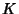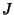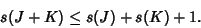## Stick Number

Let the stick numberof a Knotbe the least number of straight sticks needed to make a Knot. The smallest stick number of any Knot is, whereis the Trefoil Knot. Ifandare Knots, thenFor a nontrivial Knot, letbe the Crossing Number (i.e., the least number of crossings in any projection of). ThenThe following table gives the stick number for some common knots.